# 机器学习西瓜书学习记录-第三章 线性模型

## 第3章 线性模型

3.1基本形式

x

1

x_1

x1;

x

2

x_2

x2;…;

x

d

x_d

xd),

x

i

x_i

xi为x在第i个属性上的取值。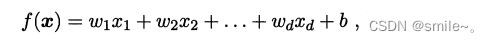w

1

w_1

w1;

w

2

w_2

w2;…;

w

d

w_d

wd) 注意是分号，故为列向量）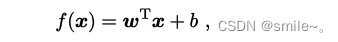w，b学得之后，模型得以确定
w直观表达了各属性在预测中的重要性
3.2线性回归
1、特殊情形-当属性数目只有一个时。
（若为离散属性，属性值间有“序”关系，可通过连续化将其转化为连续值。如三值属性"高度"的取值"高" “中” "低"可转化为 {1，0.5，0.0}；若属性值间不存在“序”关系，有k个属性值，常转化为k维向量。如属性“瓜类”取值“西瓜”“南瓜”“黄瓜”转化为(0,0,1),(0,1,0),(1,0,0)）w*， b* 表示w和b的解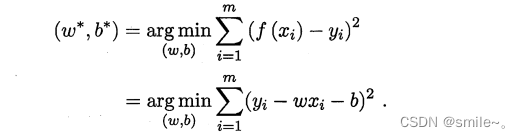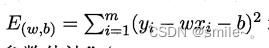E

(

w

,

b

)

E_{(w,b)}

E(w,b)分别对w，b求导得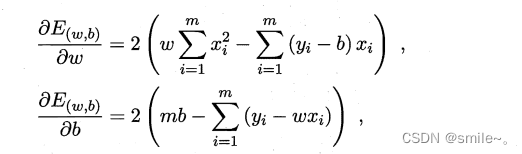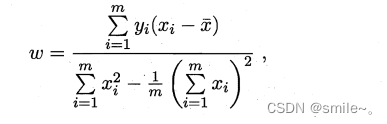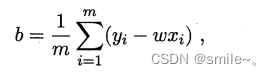2、更一般情形“多元线性回归”-样本有d个属性描述，此时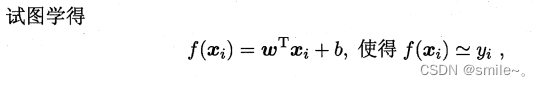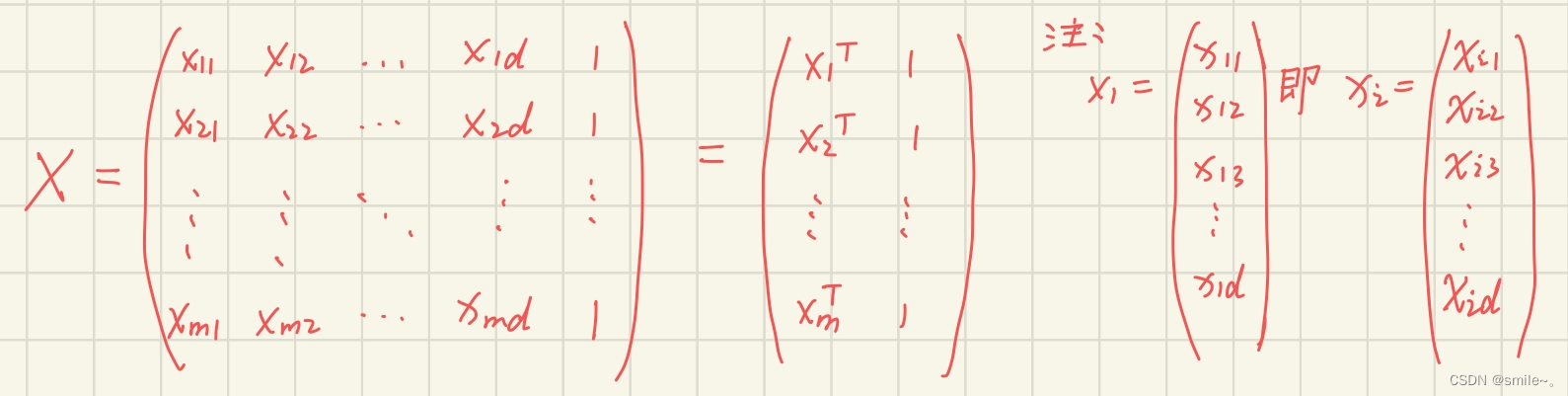w

^

=

(

w

;

b

)

\hat{w}=(w;b)

w^=(w;b)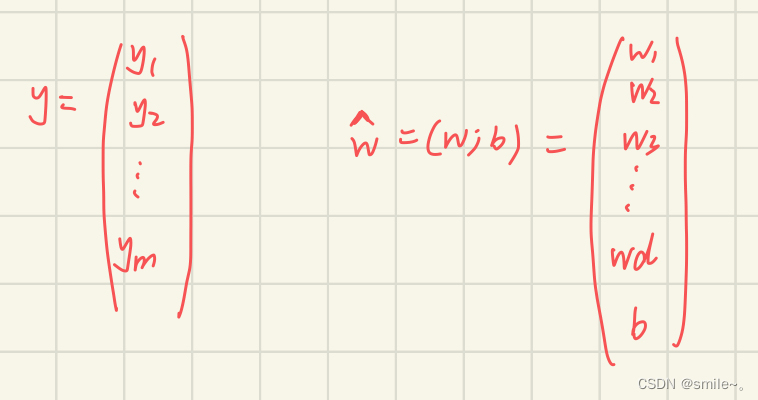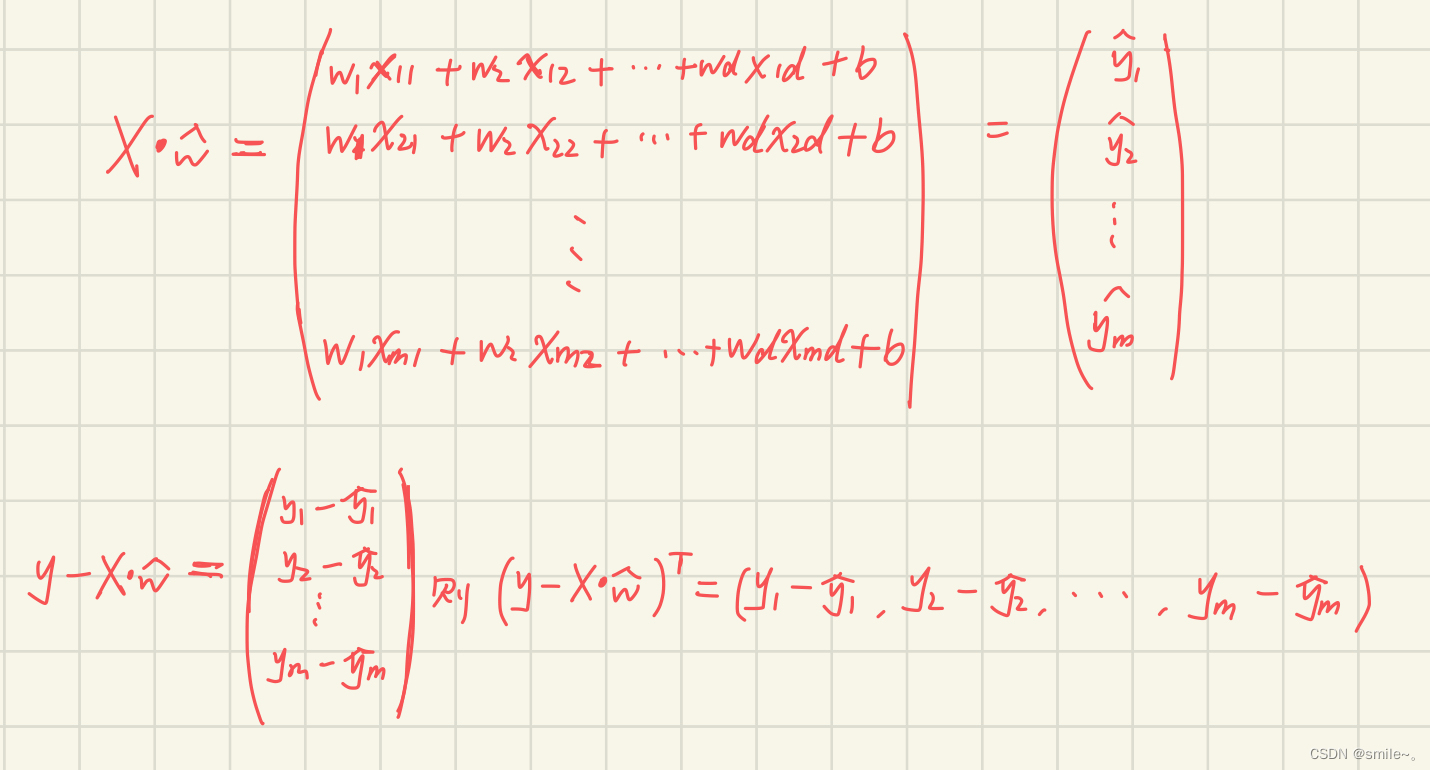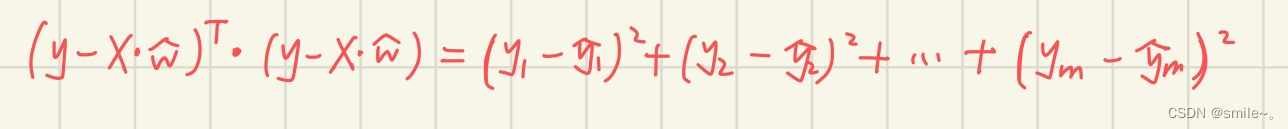w

^

=

(

w

;

b

)

\hat{w}=(w;b)

w^=(w;b)的解满足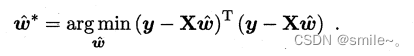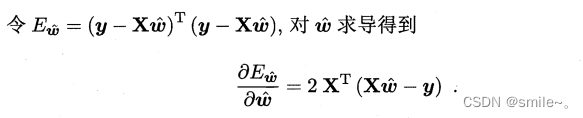w

^

\hat{w}

w^最优解的闭式解。

X

T

X

X^TX

XTX不是满秩矩阵时，可能解出多个

w

^

\hat{w}

w^，他们都可以使均方误差最小化。此时选择哪个解作为输出，由学习算法的归纳偏好决定，常见做法是引入正则化项。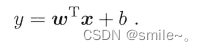假设我们认为示例所对应的输出标记是在指数尺度上变化，那就可将输出标记的对数作为线性模型逼近的目标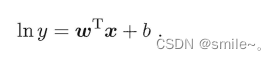e

w

T

+

b

e^{w^T+b}

ewT+b逼近y.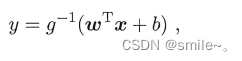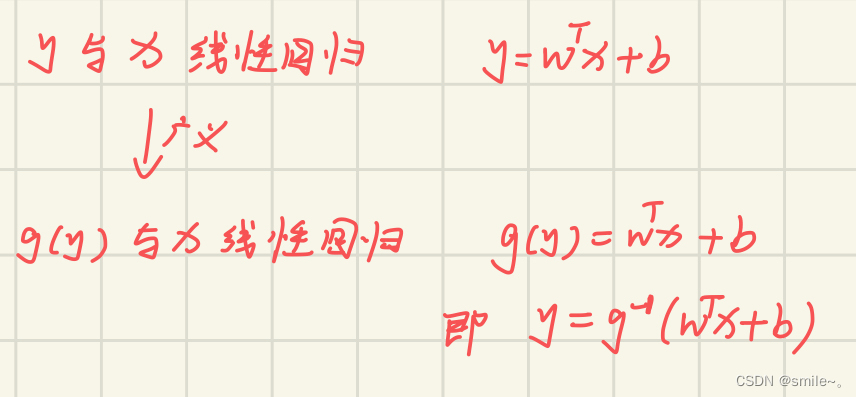3.3对数几率回归

y

{

0

,

1

}

y\in\{0,1\}

y{0,1},而线性回归模型产生预测值

z

=

w

T

x

+

b

z=w^Tx+b

z=wTx+b是实值，因此需要将实值z转换为0/1值。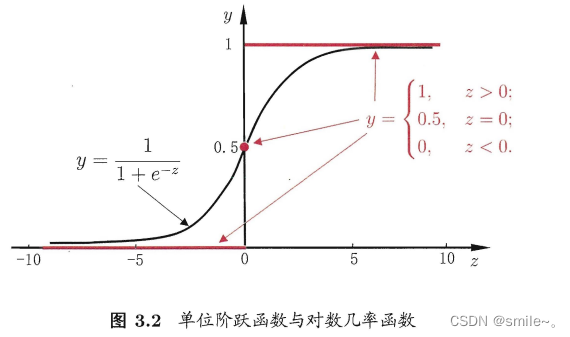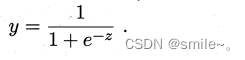z

=

w

T

x

+

b

z=w^Tx+b

z=wTx+b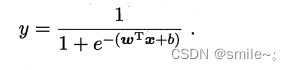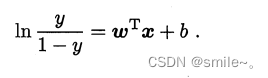y

1

y

\frac{y}{1-y}

1yy称为“几率”，反映了x作为正例的相对可能性。几率取对数得“对数几率”

l

n

y

1

y

ln\frac{y}{1-y}

ln1yy.。

3.4线性判别分析
LDA是一种经典的线性学习方法，也称“Fisher判别分析”
LDA思想：给定训练样例集，设法将样例投影到一条直线上，使得同类样例的投影点尽可能接近、异类样例的投影点尽可能远离；在对新样本进行分类时，将其投影到同样的这条直线上，再根据投影点的位置来确定新样本的类别。二维示意图如下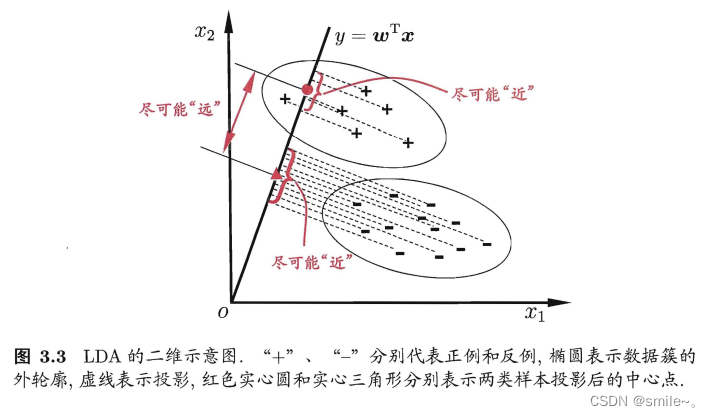3.5多分类学习

3.6类别不平衡